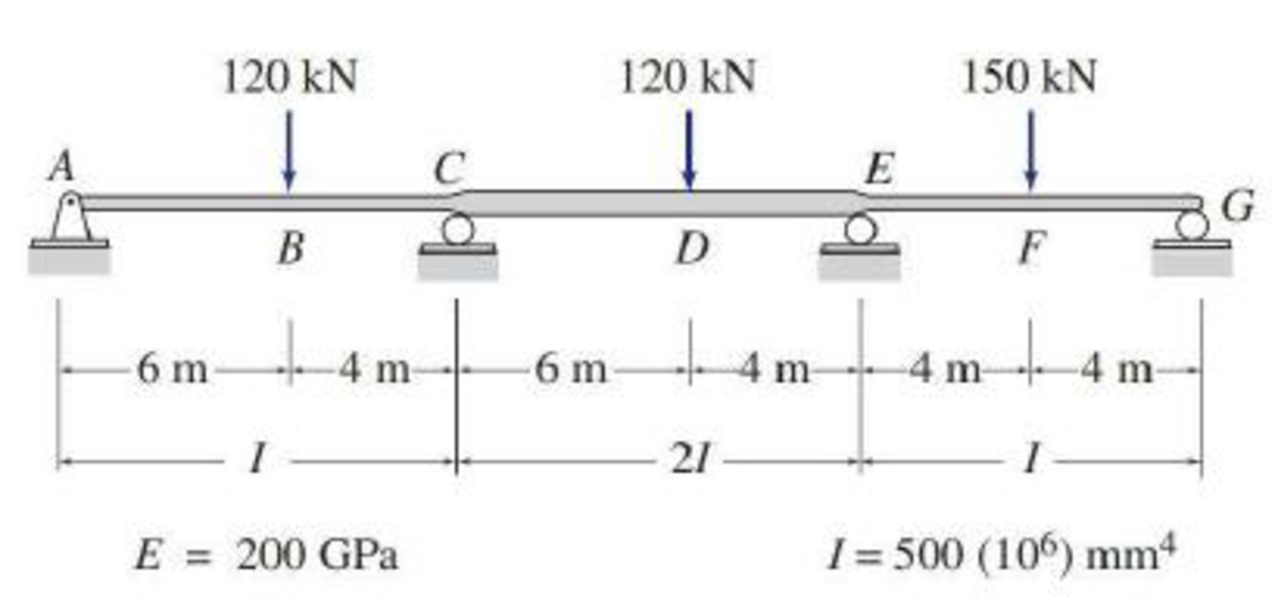# Solve Problem 16.2 for the loading shown in Fig. P16.2 and a settlement of 1 2 in. at support B. FIG. P16.12, P16.16

#### Solutions

Chapter
Section
Chapter 16, Problem 6P
Textbook Problem
118 views

## Solve Problem 16.2 for the loading shown in Fig. P16.2 and a settlement of 1 2 in. at support B.FIG. P16.12, P16.16

To determine

Find the reaction and plot the shear and bending moment diagram.

### Explanation of Solution

Fixed end moment:

Formula to calculate the relative stiffness for fixed support IL and for roller support (34)(IL).

Formula to calculate the fixed moment for point load with equal length are PL8.

Formula to calculate the fixed moment for point load with unequal length are Pab2L2 and Pa2bL2.

Formula to calculate the fixed moment for UDL is WL212.

Formula to calculate the fixed moment for deflection is 6EIΔL2

Calculation:

Show the free body diagram of the entire beam as in Figure 1.

Refer Figure 1,

Calculate the relative stiffness KBA for member AB of the beam as below:

KBA=I15+15=I30

Calculate the relative stiffness KBC for member BC of the beam as below:

KBC=I20

In the above beam, only joint B is free to rotate. Hence, calculate the distribution factor at joint C.

Calculate the distribution factor DFBA for member BA of the beam.

DFBA=KBAKBA+KBC

Substitute I30 for KBA and I20 for KBC.

DFBA=I30I30+I20=0.4

Calculate the distribution factor DFBC for member BC of the beam.

DFBC=KBCKBA+KBC

Substitute I30 for KAB and I20 for KBC.

DFBC=I20I30+I20=0.6

Check for sum of distribution factor as below:

DFBA+DFBC=1

Substitute 0.4 for DFBA and 0.6 for DFBC.

0.4+0.6=1

Hence, OK.

Refer Figure 1,

Calculate the fixed end moment for AB.

FEMAB=1.5×30212+20×308+6×29,000×1650×0.5(30ft×(12in.1ft))2=112.5+75+(1107.639kin.×1ft12in.)=279.8kft

Calculate the fixed end moment for BA.

FEMBA=1.5×3021220×308+6×29,000×1650×0.5(30ft×(12in.1ft))2=112.575+(1107.639kin.×1ft12in.)=95.2kft

Calculate the fixed end moment for BC.

FEMBC=3×202126×29,000×1650×0.5(20ft×(12in.1ft))2=100(2492.188kin.×1ft12in.)=107.7kft

Calculate the fixed end moment for CB.

FEMCB=3×202126×29,000×1650×0.5(20ft×(12in.1ft))2=100(2492.188kin.×1ft12in.)=307.7kft

Show the calculation of final moments using moment distribution method as in Table 1.

Show the section free body diagram of the member AB and BC as in Figure 2.

Consider the member AB of the beam:

Calculate the vertical reaction at the left end of the joint B by taking moment about point A.

+MA=0By,L(30)20×(15)1

### Still sussing out bartleby?

Check out a sample textbook solution.

See a sample solution

#### The Solution to Your Study Problems

Bartleby provides explanations to thousands of textbook problems written by our experts, many with advanced degrees!

Get Started

Find more solutions based on key concepts
An engineer is considering storing some radioactive material in a container she is creating. As a part of her d...

Engineering Fundamentals: An Introduction to Engineering (MindTap Course List)

bot a. compromised computer or device whose owner is unaware the computer or device is being controlled remotel...

Enhanced Discovering Computers 2017 (Shelly Cashman Series) (MindTap Course List)

The resultant of the force system shown is a 50-lbft counterclockwise couple. Find P, Q, and C.

International Edition---engineering Mechanics: Statics, 4th Edition

What two materials are most commonly used for lathe cutting tools?

Precision Machining Technology (MindTap Course List)

Why is it important to deliver effective project reports and communications?

Systems Analysis and Design (Shelly Cashman Series) (MindTap Course List)

What should be done with a leaking cylinder if the leak cannot be stopped?

Welding: Principles and Applications (MindTap Course List)

If your motherboard supports ECC DDR3 memory, can you substitute non-ECC DDR3 memory?

A+ Guide to Hardware (Standalone Book) (MindTap Course List)